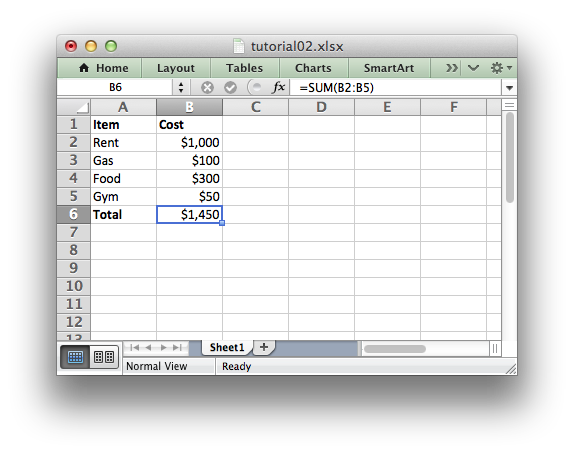libxlsxwriter
Tutorial 2: Adding formatting to the XLSX File

In the previous section we created a simple spreadsheet using C and the `libxlsxwriter` library.

This converted the required data into an Excel file but it looked a little bare. In order to make the information clearer we would like to add some simple formatting, like this:The differences here are that we have added Item and Cost column headers in a bold font, we have formatted the currency in the second column and we have made the Total string bold.

To do this we can extend our program as follows:

#include "xlsxwriter.h"
/* Some data we want to write to the worksheet. */
struct expense {
char item;
int cost;
};
struct expense expenses[] = {
{"Rent", 1000},
{"Gas", 100},
{"Food", 300},
{"Gym", 50},
};
int main() {
/* Create a workbook and add a worksheet. */
lxw_workbook *workbook = workbook_new("tutorial02.xlsx");
int row = 0;
int col = 0;
int i;
/* Add a bold format to use to highlight cells. */
/* Add a number format for cells with money. */
format_set_num_format(money, "\$#,##0");
/* Write some data header. */
worksheet_write_string(worksheet, row, col, "Item", bold);
worksheet_write_string(worksheet, row, col + 1, "Cost", bold);
/* Iterate over the data and write it out element by element. */
for (i = 0; i < 4; i++) {
/* Write from the first cell below the headers. */
row = i + 1;
worksheet_write_string(worksheet, row, col, expenses[i].item, NULL);
worksheet_write_number(worksheet, row, col + 1, expenses[i].cost, money);
}
/* Write a total using a formula. */
worksheet_write_string (worksheet, row + 1, col, "Total", bold);
worksheet_write_formula(worksheet, row + 1, col + 1, "=SUM(B2:B5)", money);
/* Save the workbook and free any allocated memory. */
return workbook_close(workbook);
}

The main difference between this and the previous program is that we have added two Format objects that we can use to format cells in the spreadsheet.

Format objects represent all of the formatting properties that can be applied to a cell in Excel such as fonts, number formatting, colors and borders. This is explained in more detail in The Format object and Working with Formats.

For now we will avoid getting into the details and just use a limited amount of the format functionality to add some simple formatting:

/* Add a bold format to use to highlight cells. */
/* Add a number format for cells with money. */
format_set_num_format(money, "\$#,##0");

We can then pass these formats as a parameter to the `worksheet_write*()` functions to format the data in the cell:

worksheet_write_string (worksheet, row + 1, col, "Total", bold);
worksheet_write_formula(worksheet, row + 1, col + 1, "=SUM(B2:B5)", money);

In the next section we will look at handling more data types.

workbook_close
lxw_error workbook_close(lxw_workbook *workbook)
Close the Workbook object and write the XLSX file.
worksheet_write_formula
lxw_error worksheet_write_formula(lxw_worksheet *worksheet, lxw_row_t row, lxw_col_t col, const char *formula, lxw_format *format)
Write a formula to a worksheet cell.
workbook_new
lxw_workbook * workbook_new(const char *filename)
Create a new workbook object.
format_set_bold
void format_set_bold(lxw_format *format)
Turn on bold for the format font.
format_set_num_format
void format_set_num_format(lxw_format *format, const char *num_format)
Set the number format for a cell.
lxw_worksheet
Struct to represent an Excel worksheet.
Definition: worksheet.h:2107
lxw_format
Struct to represent the formatting properties of an Excel format.
Definition: format.h:358
lxw_workbook
Struct to represent an Excel workbook.
Definition: workbook.h:292
worksheet_write_string
lxw_error worksheet_write_string(lxw_worksheet *worksheet, lxw_row_t row, lxw_col_t col, const char *string, lxw_format *format)
Write a string to a worksheet cell.
worksheet_write_number
lxw_error worksheet_write_number(lxw_worksheet *worksheet, lxw_row_t row, lxw_col_t col, double number, lxw_format *format)
Write a number to a worksheet cell.